# ISEE Upper Level Math : How to find an angle

## Example Questions

2 Next →

### Example Question #41 : Plane Geometry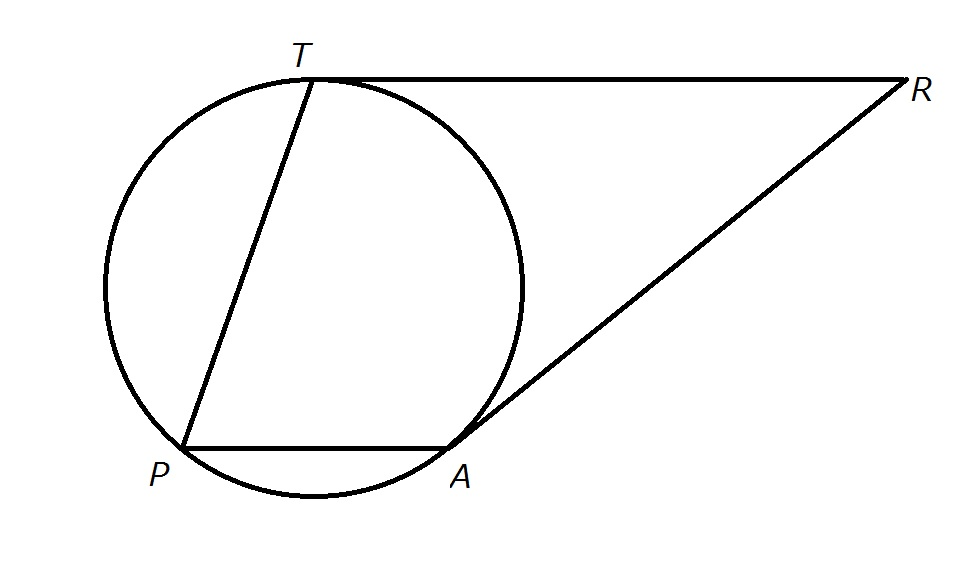Figure NOT drawn to scale

The above figure shows Trapezoid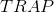, with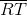and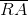tangent to the circle.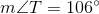; evaluate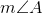.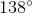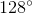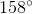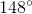Explanation:

By the Same-Side Interior Angle Theorem, since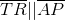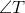and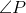are supplementary - that is, their degree measures total. Therefore,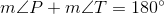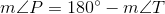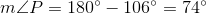is an inscribed angle, so the arc it intercepts,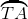, has twice its degree measure;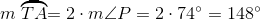.

The corresponding major arc,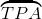, has as its measure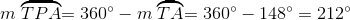The measure of an angle formed by two tangents to a circle is equal to half the difference of those of its intercepted arcs: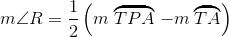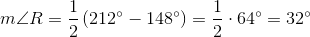Again, by the Same-Side Interior Angles Theorem,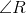and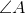are supplementary, so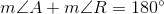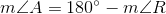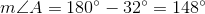2 Next →

### All ISEE Upper Level Math Resources